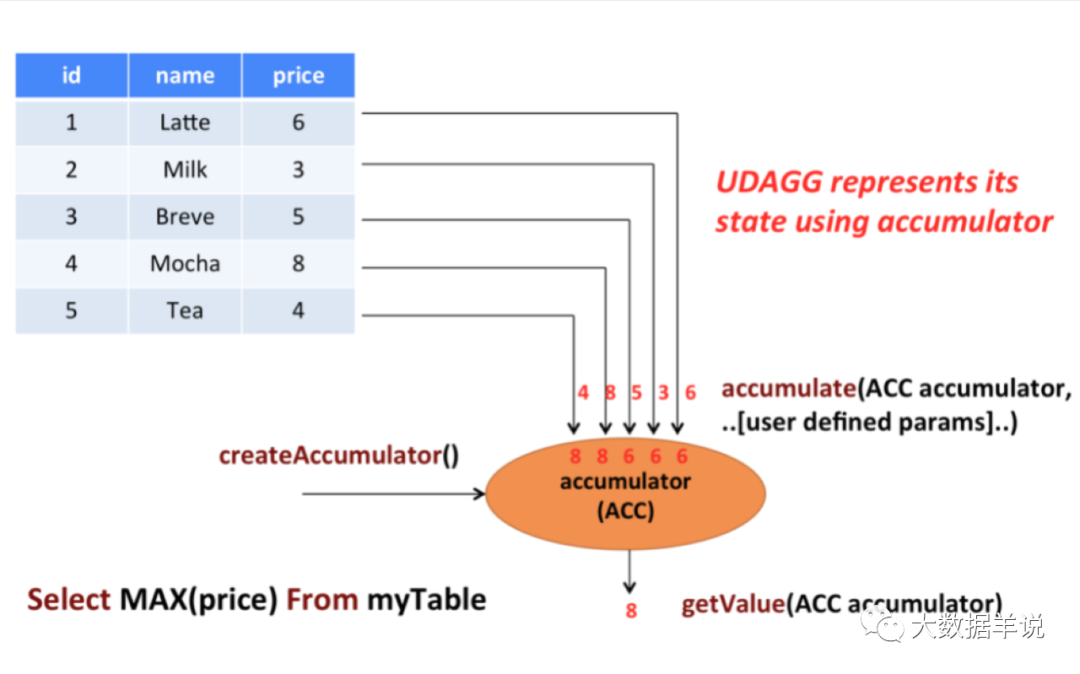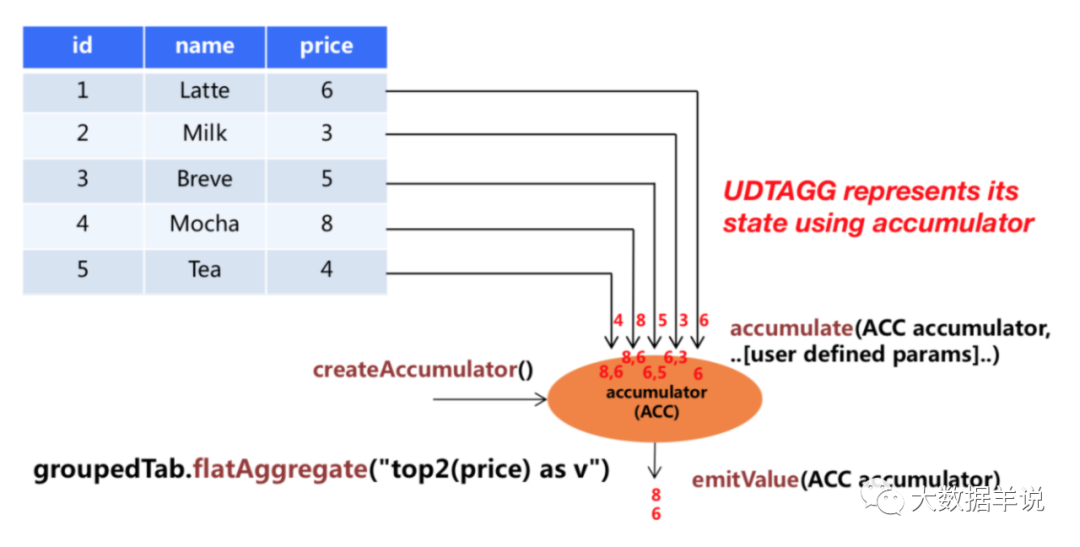crazeblue

0收藏

## 3.1SQL 函数的归类

1. ⭐ 一个归类标准是：系统（内置）函数和 Catalog 函数。系统函数没有命名空间，只能通过其名称来进行引用。Catalog 函数属于 Catalog 和数据库，因此它们拥有 Catalog 和数据库的命名空间。用户可以通过全/部分限定名（catalog.db.func 或 db.func）或者函数来对 Catalog 函数进行引用。
2. ⭐ 另一个归类标准是：临时函数和持久化函数。临时函数由用户创建，它仅在会话的生命周期（也就是一个 Flink 任务的一次运行生命周期内）内有效。持久化函数不是由系统提供的，是存储在 Catalog 中，它在不同会话的生命周期内都有效。

1. ⭐ 临时性系统内置函数
2. ⭐ 系统内置函数
3. ⭐ 临时性 Catalog 函数（例如：Create Temporary Function）
4. ⭐ Catalog 函数（例如：Create Function）

## 3.3.SQL 函数的解析顺序

### 3.3.1.精确函数

1. ⭐ 临时性 catalog 函数
2. ⭐ Catalog 函数

### 3.3.2.模糊函数

1. ⭐ 临时性系统内置函数
2. ⭐ 系统内置函数
3. ⭐ 临时性 Catalog 函数, 只会在当前会话的当前 Catalog 和当前数据库中查找函数及解析函数
4. ⭐ Catalog 函数, 在当前 Catalog 和当前数据库中查找函数及解析函数

## 3.5.SQL 自定义函数（UDF）

1. 标量函数（Scalar functions 或​`​UDAF​`​）：输入一条输出一条，将标量值转换成一个新标量值，对标 Hive 中的 UDF；
2. 表值函数（Table functions 或​`​UDTF​`​）：输入一条条输出多条，对标 Hive 中的 UDTF；
3. 聚合函数（Aggregate functions 或​`​UDAF​`​）：输入多条输出一条，对标 Hive 中的 UDAF；
4. 表值聚合函数（Table aggregate functions 或​`​UDTAF​`​）：仅仅支持 Table API，不支持 SQL API，其可以将多行转为多行；
5. 异步表值函数（Async table functions）：这是一种特殊的 UDF，支持异步查询外部数据系统，用在前文介绍到的 lookup join 中作为查询外部系统的函数。

``````import org.apache.flink.table.api.*;

// 定义一个标量函数
public static class SubstringFunction extends ScalarFunction {
public String eval(String s, Integer begin, Integer end){
return s.substring(begin, end);
}
}

TableEnvironment env = TableEnvironment.create(...);

// 在 Table API 可以直接以引用 class 方式使用 UDF
env.from("MyTable").select(call(SubstringFunction.class, \$("myField"), 5, 12));

// 注册 UDF
env.createTemporarySystemFunction("SubstringFunction", SubstringFunction.class);

// Table API 调用 UDF
env.from("MyTable").select(call("SubstringFunction", \$("myField"), 5, 12));

// SQL API 调用 UDF
env.sqlQuery("SELECT SubstringFunction(myField, 5, 12) FROM MyTable");``````

``````import org.apache.flink.table.api.*;

// 定义一个带有输入参数的标量函数
public static class SubstringFunction extends ScalarFunction {

-- boolean 默认就是 Serializable 的
private boolean endInclusive;

public SubstringFunction(boolean endInclusive){
this.endInclusive = endInclusive;
}

public String eval(String s, Integer begin, Integer end){
return s.substring(begin, endInclusive ? end + 1 : end);
}
}

TableEnvironment env = TableEnvironment.create(...);

// Table API 调用 UDF
env.from("MyTable").select(call(new SubstringFunction(true), \$("myField"), 5, 12));

// 注册 UDF
env.createTemporarySystemFunction("SubstringFunction", new SubstringFunction(true));``````

## 3.6.开发 UDF 之前的需知事项

1. 首先需要继承 Flink SQL UDF 体系提供的基类，每种 UDF 实现都有不同的基类
2. 实现 UDF 执行逻辑函数，不同类型的 UDF 需要实现不同的执行逻辑函数
5. 巧妙运用运行时上下文，可以在任务运行前加载到一些外部资源、上下文配置信息，扩展 UDF 能力

### 3.6.1.继承 UDF 基类

1. ⭐ Flink UDF 要继承一个基类（比如标量 UDF 要继承​`​org.apache.flink.table.functions.ScalarFunction​`​）。
2. ⭐ 类必须声明为​`​public​`​​，不能是​`​abstract​`​ 类，不能使用非静态内部类或匿名类。
3. ⭐ 为了在 Catalog 中存储此类，该类必须要有默认构造函数并且在运行时可以进行实例化。

### 3.6.2.实现 UDF 执行逻辑函数

1. ⭐​`​open()​`​：用于初始化资源（比如连接外部资源），程序初始化时进行调用
2. ⭐​`​close()​`​：用于关闭资源，程序结束时进行调用
3. ⭐​`​isDeterministic()​`​：用于判断返回结果是否是确定的，如果是确定的，结果会被直接执行
4. ⭐​`​eval(xxx)​`​：Flink 用于处理每一条数据的主要处理逻辑函数

• eval(Integer) 和 eval(LocalDateTime)；
• 使用变长参数，例如 eval(Integer...);
• 使用对象，例如 eval(Object) 可接受 LocalDateTime、Integer 作为参数，只要是 Object 都可以；
• 也可组合使用，例如 eval(Object...) 可接受所有类型的参数。

``````import org.apache.flink.table.functions.ScalarFunction;

// 有多个重载求和方法的函数
public static class SumFunction extends ScalarFunction {

// 入参为 Integer
public Integer eval(Integer a, Integer b){
return a + b;
}

// 入参为 String
public Integer eval(String a, String b){
return Integer.valueOf(a) + Integer.valueOf(b);
}

// 入参为多个 Double
public Integer eval(Double... d){
double result = 0;
for (double value : d)
result += value;
return (int) result;
}
}``````

### 3.6.3.注意 UDF 入参、出参类型推导

1. ⭐ 从开发人员角度讲，在设计 UDF 的时候，肯定会涉及到 UDF 预期的入参、出参类型信息、也包括一些数据的精度、小数位数等信息
2. ⭐ 从程序运行角度讲，Flink SQL 程序运行时，肯定也需要知道怎么将 SQL 中的类型数据与 UDF 的入参、出参类型，这样才能做数据序列化等操作

2. ⭐ 添加类型注解：当 1 中的隐式反射提取方法不成功，则可以通过使用 Flink 提供的​`​@DataTypeHint​`​​ 和​`​@FunctionHint​`​ 注解对应的参数、类或方法来显示的支持 Flink 参数类型提取。
3. ⭐ 重写​`​getTypeInference()​`​​：你可以使用 Flink 提供的更高级的类型推导方法，你可以在 UDF 实现类中重写​`​getTypeInference()​`​ 方法去显示声明函数的参数类型信息

1. ⭐ 自动类型推导案例：

``````import org.apache.flink.table.annotation.DataTypeHint;

// 有多个重载求值方法的函数
public static class OverloadedFunction extends ScalarFunction {

// 不需要任何声明，可以直接推导出类型信息，即入参和出参对应到 SQL 中的 bigint 类型
public Long eval(long a, long b){
return a + b;
}

// 使用 @DataTypeHint("DECIMAL(12, 3)") 定义 decimal 的精度和小数位
public @DataTypeHint("DECIMAL(12, 3)") BigDecimal eval(double a, double b){
return BigDecimal.valueOf(a + b);
}

// 使用注解定义嵌套数据类型
@DataTypeHint("ROW<s STRING, t TIMESTAMP_LTZ(3)>")
public Row eval(int i){
return Row.of(String.valueOf(i), Instant.ofEpochSecond(i));
}

// 允许任意类型的输入，并输出序列化定制后的值
@DataTypeHint(value = "RAW", bridgedTo = ByteBuffer.class)
public ByteBuffer eval(@DataTypeHint(inputGroup = InputGroup.ANY) Object o) {
return MyUtils.serializeToByteBuffer(o);
}
}``````
1. ⭐ 根据 @FunctionHint 注解自动推导类型案例：

• ⭐ 我们不希望 eval 函数的入参和出参都是一个非常具体的类型，比如 long，int，double 等。我们希望它是一个通用的类型，比如 Object。这样的话就不用重载那么多的函数，可以直接使用一个 eval 函数实现不同的处理逻辑，返回不同类型的结果
• ⭐ 多个 eval 方法的返回结果类型都是相同的，我们懒得写多次 @DataTypeHint

``````import org.apache.flink.table.annotation.DataTypeHint;

// 1. 解耦类型推导与 eval 方法，类型推导根据 FunctionHint 注解中的信息来，下面的案例说明当前这个 UDF 有三种输入输出类型信息组合
@FunctionHint(
input = {@DataTypeHint("INT"), @DataTypeHint("INT")},
output = @DataTypeHint("INT")
)
@FunctionHint(
input = {@DataTypeHint("BIGINT"), @DataTypeHint("BIGINT")},
output = @DataTypeHint("BIGINT")
)
@FunctionHint(
input = {},
output = @DataTypeHint("BOOLEAN")
)
public static class OverloadedFunction extends TableFunction<Object> {

public void eval(Object... o){
if (o.length == 0) {
collect(false);
}
collect(o);
}
}

// 2. 为函数类的所有 eval 方法指定同一个输出类型
@FunctionHint(output = @DataTypeHint("ROW<s STRING, i INT>"))
public static class OverloadedFunction extends TableFunction<Row> {

public void eval(int a, int b){
collect(Row.of("Sum", a + b));
}

public void eval(){
collect(Row.of("Empty args", -1));
}
}``````
1. ⭐ getTypeInference()

getTypeInference() 可以做到根据小伙伴萌自定义的方式去定义类型推导过程及结果，具有高度自定义的能力。举例如下：

``````import org.apache.flink.table.api.DataTypes;

public static class LiteralFunction extends ScalarFunction {
public Object eval(String s, String type){
switch (type) {
case "INT":
return Integer.valueOf(s);
case "DOUBLE":
return Double.valueOf(s);
case "STRING":
default:
return s;
}
}

// 如果实现了 getTypeInference，则会禁用自动的反射式类型推导，使用如下逻辑进行类型推导
@Override
public TypeInference getTypeInference(DataTypeFactory typeFactory){
return TypeInference.newBuilder()
// 指定输入参数的类型，必要时参数会被隐式转换
.typedArguments(DataTypes.STRING(), DataTypes.STRING())
// 用户高度自定义的类型推导逻辑
.outputTypeStrategy(callContext -> {
if (!callContext.isArgumentLiteral(1) || callContext.isArgumentNull(1)) {
throw callContext.newValidationError("Literal expected for second argument.");
}
// 基于第一个入参决定具体的返回数据类型
final String literal = callContext.getArgumentValue(1, String.class).orElse("STRING");
switch (literal) {
case "INT":
return Optional.of(DataTypes.INT().notNull());
case "DOUBLE":
return Optional.of(DataTypes.DOUBLE().notNull());
case "STRING":
default:
return Optional.of(DataTypes.STRING());
}
})
.build();
}
}``````

### 3.6.4.明确 UDF 输出结果是否是定值

1. ⭐ Flink 在生成计划期间直接执行 UDF 获得结果：如果使用常量表达式调用函数，或者使用常量作为函数的入参，则 Flink 任务可能不会在任务正式运行时执行该函数。举个例子，​`​SELECT ABS(-1) FROM t​`​​，​`​SELECT ABS(field) FROM t WHERE field = -1​`​​，这两种都会被 Flink 进行优化，直接把 ABS(-1) 的结果在客户端生成执行计划时就将结果运行出来。如果不想在生成执行计划阶段直接将结果运行出来，可以实现​`​isDeterministic()​`​ 返回 false。
2. ⭐ Flink 在程序运行期间执行 UDF 获得结果：如果 UDF 的入参不是常量表达式，或者​`​isDeterministic()​`​ 返回 false，则 Flink 会在程序运行期间执行 UDF。

### 3.6.5.巧妙运用运行时上下文

1. ⭐​`​open()​`​ 方法：在任务初始化时被调用，常常用于加载一些外部资源；
2. ⭐​`​close()​`​ 方法：在任务结束时被调用，常常用于关闭一些外部资源；

1. ⭐ getMetricGroup()：执行该函数的 subtask 的 Metric Group
2. ⭐ getCachedFile(name)：分布式文件缓存的本地临时文件副本
3. ⭐ getJobParameter(name, defaultValue)：获取 Flink 任务的全局作业参数
4. ⭐ getExternalResourceInfos(resourceName)：获取一些外部资源

``````import org.apache.flink.table.api.*;

public static class HashCodeFunction extends ScalarFunction {

private int factor = 0;

@Override
public void open(FunctionContext context) throws Exception {
// 4. 在 UDF 中获取全局参数 hashcode_factor
// 用户可以配置全局作业参数 "hashcode_factor"
// 获取参数 "hashcode_factor"
// 如果不存在，则使用默认值 "12"
factor = Integer.parseInt(context.getJobParameter("hashcode_factor", "12"));
}

public int eval(String s){
return s.hashCode() * factor;
}
}

TableEnvironment env = TableEnvironment.create(...);

// 1. 设置任务参数

// 2. 注册函数
env.createTemporarySystemFunction("hashCode", HashCodeFunction.class);

// 3. 调用函数
env.sqlQuery("SELECT myField, hashCode(myField) FROM MyTable");``````

## 3.7.SQL 标量函数（Scalar Function）

1. ⭐ 实现​`​org.apache.flink.table.functions.ScalarFunction​`​ 接口
2. ⭐ 实现一个或者多个自定义的 eval 函数，名称必须叫做 eval，eval 方法签名必须是 public 的
3. ⭐ eval 方法的入参、出参都是直接体现在 eval 函数的签名中

``````import org.apache.flink.table.annotation.InputGroup;

public static class HashFunction extends ScalarFunction {

// 接受任意类型输入，返回 INT 型输出
public int eval(@DataTypeHint(inputGroup = InputGroup.ANY){
return o.hashCode();
}
}

TableEnvironment env = TableEnvironment.create(...);

// 在 Table API 里不经注册直接调用函数
env.from("MyTable").select(call(HashFunction.class, \$("myField")));

// 注册函数
env.createTemporarySystemFunction("HashFunction", HashFunction.class);

// 在 Table API 里调用注册好的函数
env.from("MyTable").select(call("HashFunction", \$("myField")));

// 在 SQL 里调用注册好的函数
env.sqlQuery("SELECT HashFunction(myField) FROM MyTable");``````

## 3.8.SQL 表值函数（Table Function）

1. ⭐ 实现​`​org.apache.flink.table.functions.TableFunction​`​ 接口
2. ⭐ 实现一个或者多个自定义的 eval 函数，名称必须叫做 eval，eval 方法签名必须是 public 的
3. ⭐ eval 方法的入参是直接体现在 eval 函数签名中，出参是体现在 TableFunction 类的泛型参数 T 中，eval 是没有返回值的，这一点是和标量函数不同的，Flink TableFunction 接口提供了​`​collect(T)​`​​ 来发送输出的数据。这一点也比较好理解，如果都体现在函数签名上，那就成了标量函数了，而使用​`​collect(T)​`​​ 才能体现出​`​进一条数据​`​​`​出多条数据​`

``````import org.apache.flink.table.annotation.DataTypeHint;

@FunctionHint(output = @DataTypeHint("ROW<word STRING, length INT>"))
public static class SplitFunction extends TableFunction<Row> {

public void eval(String str){
for (String s : str.split(" ")) {
// 输出结果
collect(Row.of(s, s.length()));
}
}
}

TableEnvironment env = TableEnvironment.create(...);

// 在 Table API 里可以直接调用 UDF
env
.from("MyTable")
.joinLateral(call(SplitFunction.class, \$("myField")))
.select(\$("myField"), \$("word"), \$("length"));

env
.from("MyTable")
.leftOuterJoinLateral(call(SplitFunction.class, \$("myField")))
.select(\$("myField"), \$("word"), \$("length"));

// 在 Table API 里重命名 UDF 的结果字段
env
.from("MyTable")
.leftOuterJoinLateral(call(SplitFunction.class, \$("myField")).as("newWord", "newLength"))
.select(\$("myField"), \$("newWord"), \$("newLength"));

// 注册函数
env.createTemporarySystemFunction("SplitFunction", SplitFunction.class);

// 在 Table API 里调用注册好的 UDF
env
.from("MyTable")
.joinLateral(call("SplitFunction", \$("myField")))
.select(\$("myField"), \$("word"), \$("length"));

env
.from("MyTable")
.leftOuterJoinLateral(call("SplitFunction", \$("myField")))
.select(\$("myField"), \$("word"), \$("length"));

// 在 SQL 里调用注册好的 UDF
env.sqlQuery(
"SELECT myField, word, length " +
"FROM MyTable, LATERAL TABLE(SplitFunction(myField))");

env.sqlQuery(
"SELECT myField, word, length " +
"FROM MyTable " +
"LEFT JOIN LATERAL TABLE(SplitFunction(myField)) ON TRUE");

// 在 SQL 里重命名 UDF 字段
env.sqlQuery(
"SELECT myField, newWord, newLength " +
"FROM MyTable " +
"LEFT JOIN LATERAL TABLE(SplitFunction(myField)) AS T(newWord, newLength) ON TRUE");``````

## 3.9.SQL 聚合函数（Aggregate Function）UDAF

1. ⭐ 实现​`​AggregateFunction​`​ 接口，其中所有的方法必须是 public 的、非 static 的
2. ⭐ 必须实现以下几个方法：
• ⭐​`​Acc聚合中间结果 createAccumulator()​`​：为当前 Key 初始化一个空的 accumulator，其存储了聚合的中间结果，比如在执行 max() 时会存储当前的 max 值
• ⭐​`​accumulate(Acc accumulator, Input输入参数)​`​：对于每一行数据，都会调用 accumulate() 方法来更新 accumulator，这个方法就是用于处理每一条输入数据；这个方法必须声明为 public 和非 static 的。accumulate 方法可以重载，每个方法的参数类型可以不同，并且支持变长参数。
• ⭐​`​Output输出参数 getValue(Acc accumulator)​`​：通过调用 getValue 方法来计算和返回最终的结果
1. ⭐ 还有几个方法是在某些场景下才必须实现的：
• ⭐​`​retract(Acc accumulator, Input输入参数)​`​：在回撤流的场景下必须要实现，Flink 在计算回撤数据时需要进行调用，如果没有实现则会直接报错
• ⭐​`​merge(Acc accumulator, Iterable<Acc> it)​`​：在许多批式聚合以及流式聚合中的 Session、Hop 窗口聚合场景下都是必须要实现的。除此之外，这个方法对于优化也很多帮助。例如，如果你打开了两阶段聚合优化，就需要 AggregateFunction 实现 merge 方法，从而可以做到在数据进行 shuffle 前先进行一次聚合计算。
• ⭐​`​resetAccumulator()​`​：在批式聚合中是必须实现的。
1. ⭐ 还有几个关于入参、出参数据类型信息的方法，默认情况下，用户的​`​Input输入参数​`​​（​`​accumulate(Acc accumulator, Input输入参数)​`​​ 的入参​`​Input输入参数​`​​）、accumulator（​`​Acc聚合中间结果 createAccumulator()​`​​ 的返回结果）、​`​Output输出参数​`​​ 数据类型（​`​Output输出参数 getValue(Acc accumulator)​`​​ 的​`​Output输出参数​`​​）都会被 Flink 使用反射获取到。但是对于​`​accumulator​`​​ 和​`​Output输出参数​`​​ 类型来说，Flink SQL 的类型推导在遇到复杂类型的时候可能会推导出错误的结果（注意：​`​Input输入参数​`​​ 因为是上游算子传入的，所以类型信息是确认的，不会出现推导错误的情况），比如那些非基本类型 POJO 的复杂类型。所以跟 ScalarFunction 和 TableFunction 一样，AggregateFunction 提供了​`​AggregateFunction#getResultType()​`​​ 和​`​AggregateFunction#getAccumulatorType()​`​ 来分别指定最终返回值类型和 accumulator 的类型，两个函数的返回值类型都是 TypeInformation，所以熟悉 DataStream 的小伙伴很容易上手。
• ⭐​`​getResultType()​`​​：即​`​Output输出参数 getValue(Acc accumulator)​`​ 的输出结果数据类型
• ⭐​`​getAccumulatorType()​`​​：即​`​Acc聚合中间结果 createAccumulator()​`​ 的返回结果数据类型

• ⭐ 定义一个聚合函数来计算某一列的加权平均
• ⭐ 在 TableEnvironment 中注册函数
• ⭐ 在查询中使用函数

``````import org.apache.flink.table.api.*;

// 自定义一个计算权重 avg 的 accmulator
public static class WeightedAvgAccumulator {
public long sum = 0;
public int count = 0;
}

// 输入：Long iValue, Integer iWeight
public static class WeightedAvg extends AggregateFunction<Long, WeightedAvgAccumulator> {

@Override
// 创建一个 accumulator
public WeightedAvgAccumulator createAccumulator(){
return new WeightedAvgAccumulator();
}

public void accumulate(WeightedAvgAccumulator acc, Long iValue, Integer iWeight){
acc.sum += iValue * iWeight;
acc.count += iWeight;
}

public void retract(WeightedAvgAccumulator acc, Long iValue, Integer iWeight){
acc.sum -= iValue * iWeight;
acc.count -= iWeight;
}

@Override
// 获取返回结果
public Long getValue(WeightedAvgAccumulator acc){
if (acc.count == 0) {
return null;
} else {
return acc.sum / acc.count;
}
}

// Session window 可以使用这个方法将几个单独窗口的结果合并
public void merge(WeightedAvgAccumulator acc, Iterable<WeightedAvgAccumulator> it){
for (WeightedAvgAccumulator a : it) {
acc.count += a.count;
acc.sum += a.sum;
}
}

public void resetAccumulator(WeightedAvgAccumulator acc){
acc.count = 0;
acc.sum = 0L;
}
}

TableEnvironment env = TableEnvironment.create(...);

env
.from("MyTable")
.groupBy(\$("myField"))
.select(\$("myField"), call(WeightedAvg.class, \$("value"), \$("weight")));

// 注册函数
env.createTemporarySystemFunction("WeightedAvg", WeightedAvg.class);

// Table API 调用函数
env
.from("MyTable")
.groupBy(\$("myField"))
.select(\$("myField"), call("WeightedAvg", \$("value"), \$("weight")));

// SQL API 调用函数
env.sqlQuery(
"SELECT myField, WeightedAvg(`value`, weight) FROM MyTable GROUP BY myField"
);``````

## 3.10.SQL 表值聚合函数（Table Aggregate Function）UDTAF

1. ⭐ 实现​`​TableAggregateFunction​`​ 接口，其中所有的方法必须是 public 的、非 static 的
2. ⭐ 必须实现以下几个方法：
• ⭐​`​Acc聚合中间结果 createAccumulator()​`​：为当前 Key 初始化一个空的 accumulator，其存储了聚合的中间结果，比如在执行 max() 时会存储每一条中间结果的 max 值
• ⭐​`​accumulate(Acc accumulator, Input输入参数)​`​：对于每一行数据，都会调用 accumulate() 方法来更新 accumulator，这个方法就是对每一条输入数据进行执行，比如执行 max() 时，遍历每一条数据执行；在实现这个方法是必须声明为 public 和非 static 的。accumulate 方法可以重载，每个方法的参数类型不同，并且支持变长参数。
• ⭐​`​emitValue(Acc accumulator, Collector<OutPut> collector)​`​​ 或者​`​emitUpdateWithRetract(Acc accumulator, RetractableCollector<OutPut> collector)​`​：当遍历所有的数据，当所有的数据都处理完了之后，通过调用 emit 方法来计算和输出最终的结果，在这里你就可以自定义到底输出多条少以及怎么样去输出结果。那么对于 emitValue 以及 emitUpdateWithRetract 的区别来说，拿 TopN 实现来说，emitValue 每次都会发送所有的最大的 n 个值，而这在流式任务中可能会有一些性能问题。为了提升性能，用户可以实现 emitUpdateWithRetract 方法。这个方法在 retract 模式下会增量的输出结果，比如只在有数据更新时，可以做到撤回老的数据，然后再发送新的数据，而不需要每次都发出全量的最新数据。如果我们同时定义了 emitUpdateWithRetract、emitValue 方法，那 emitUpdateWithRetract 会优先于 emitValue 方法被使用，因为引擎会认为 emitUpdateWithRetract 会更加高效，因为它的输出是增量的。
1. ⭐ 还有几个方法是在某些场景下才必须实现的：
• ⭐​`​retract(Acc accumulator, Input输入参数)​`​：在回撤流的场景下必须要实现，Flink 在计算回撤数据时需要进行调用，如果没有实现则会直接报错
• ⭐​`​merge(Acc accumulator, Iterable<Acc> it)​`​：在许多批式聚合以及流式聚合中的 Session、Hop 窗口聚合场景下都是必须要实现的。除此之外，这个方法对于优化也很多帮助。例如，如果你打开了两阶段聚合优化，就需要 AggregateFunction 实现 merge 方法，从而在第一阶段先进行数据聚合。
• ⭐​`​resetAccumulator()​`​：在批式聚合中是必须实现的。
1. ⭐ 还有几个关于入参、出参数据类型信息的方法，默认情况下，用户的​`​Input输入参数​`​​（​`​accumulate(Acc accumulator, Input输入参数)​`​​ 的入参​`​Input输入参数​`​​）、accumulator（​`​Acc聚合中间结果 createAccumulator()​`​​ 的返回结果）、​`​Output输出参数​`​​ 数据类型（​`​emitValue(Acc acc, Collector<Output输出参数> out)​`​​ 的​`​Output输出参数​`​​）都会被 Flink 使用反射获取到。但是对于​`​accumulator​`​​ 和​`​Output输出参数​`​​ 类型来说，Flink SQL 的类型推导在遇到复杂类型的时候可能会推导出错误的结果（注意：​`​Input输入参数​`​​ 因为是上游算子传入的，所以类型信息是确认的，不会出现推导错误的情况），比如那些非基本类型 POJO 的复杂类型。所以跟 ScalarFunction 和 TableFunction 一样，AggregateFunction 提供了​`​TableAggregateFunction#getResultType()​`​​ 和​`​TableAggregateFunction#getAccumulatorType()​`​ 来分别指定最终返回值类型和 accumulator 的类型，两个函数的返回值类型都是 TypeInformation，所以熟悉 DataStream 的小伙伴很容易上手。
• ⭐​`​getResultType()​`​​：即​`​emitValue(Acc acc, Collector<Output输出参数> out)​`​ 的输出结果数据类型
• ⭐​`​getAccumulatorType()​`​​：即​`​Acc聚合中间结果 createAccumulator()​`​ 的返回结果数据类型

• ⭐ 定义一个 TableAggregateFunction 来计算给定列的最大的 2 个值
• ⭐ 在 TableEnvironment 中注册函数
• ⭐ 在 Table API 查询中使用函数（当前只在 Table API 中支持 TableAggregateFunction）

``````/**
* Accumulator for Top2.
*/
public class Top2Accum {
public Integer first;
public Integer second;
}

public static class Top2 extends TableAggregateFunction<Tuple2<Integer, Integer>, Top2Accum> {

@Override
public Top2Accum createAccumulator(){
Top2Accum acc = new Top2Accum();
acc.first = Integer.MIN_VALUE;
acc.second = Integer.MIN_VALUE;
return acc;
}

public void accumulate(Top2Accum acc, Integer v){
if (v > acc.first) {
acc.second = acc.first;
acc.first = v;
} else if (v > acc.second) {
acc.second = v;
}
}

public void merge(Top2Accum acc, java.lang.Iterable<Top2Accum> iterable){
for (Top2Accum otherAcc : iterable) {
accumulate(acc, otherAcc.first);
accumulate(acc, otherAcc.second);
}
}

public void emitValue(Top2Accum acc, Collector<Tuple2<Integer, Integer>> out){
// emit the value and rank
if (acc.first != Integer.MIN_VALUE) {
out.collect(Tuple2.of(acc.first, 1));
}
if (acc.second != Integer.MIN_VALUE) {
out.collect(Tuple2.of(acc.second, 2));
}
}
}

// 注册函数
StreamTableEnvironment tEnv = ...
tEnv.registerFunction("top2", new Top2());

// 初始化表
Table tab = ...;

// 使用函数
tab.groupBy("key")
.flatAggregate("top2(a) as (v, rank)")
.select("key, v, rank");``````

``````/**
* Accumulator for Top2.
*/
public class Top2Accum {
public Integer first;
public Integer second;
public Integer oldFirst;
public Integer oldSecond;
}

public static class Top2 extends TableAggregateFunction<Tuple2<Integer, Integer>, Top2Accum> {

@Override
public Top2Accum createAccumulator(){
Top2Accum acc = new Top2Accum();
acc.first = Integer.MIN_VALUE;
acc.second = Integer.MIN_VALUE;
acc.oldFirst = Integer.MIN_VALUE;
acc.oldSecond = Integer.MIN_VALUE;
return acc;
}

public void accumulate(Top2Accum acc, Integer v){
if (v > acc.first) {
acc.second = acc.first;
acc.first = v;
} else if (v > acc.second) {
acc.second = v;
}
}

public void emitUpdateWithRetract(Top2Accum acc, RetractableCollector<Tuple2<Integer, Integer>> out){
if (!acc.first.equals(acc.oldFirst)) {
// if there is an update, retract old value then emit new value.
if (acc.oldFirst != Integer.MIN_VALUE) {
out.retract(Tuple2.of(acc.oldFirst, 1));
}
out.collect(Tuple2.of(acc.first, 1));
acc.oldFirst = acc.first;
}

if (!acc.second.equals(acc.oldSecond)) {
// if there is an update, retract old value then emit new value.
if (acc.oldSecond != Integer.MIN_VALUE) {
out.retract(Tuple2.of(acc.oldSecond, 2));
}
out.collect(Tuple2.of(acc.second, 2));
acc.oldSecond = acc.second;
}
}
}

// 注册函数
StreamTableEnvironment tEnv = ...
tEnv.registerFunction("top2", new Top2());

// 初始化表
Table tab = ...;

// 使用函数
tab.groupBy("key")
.flatAggregate("top2(a) as (v, rank)")
.select("key, v, rank");``````帖子
视频
声望
粉丝
最近发布
社区精华内容